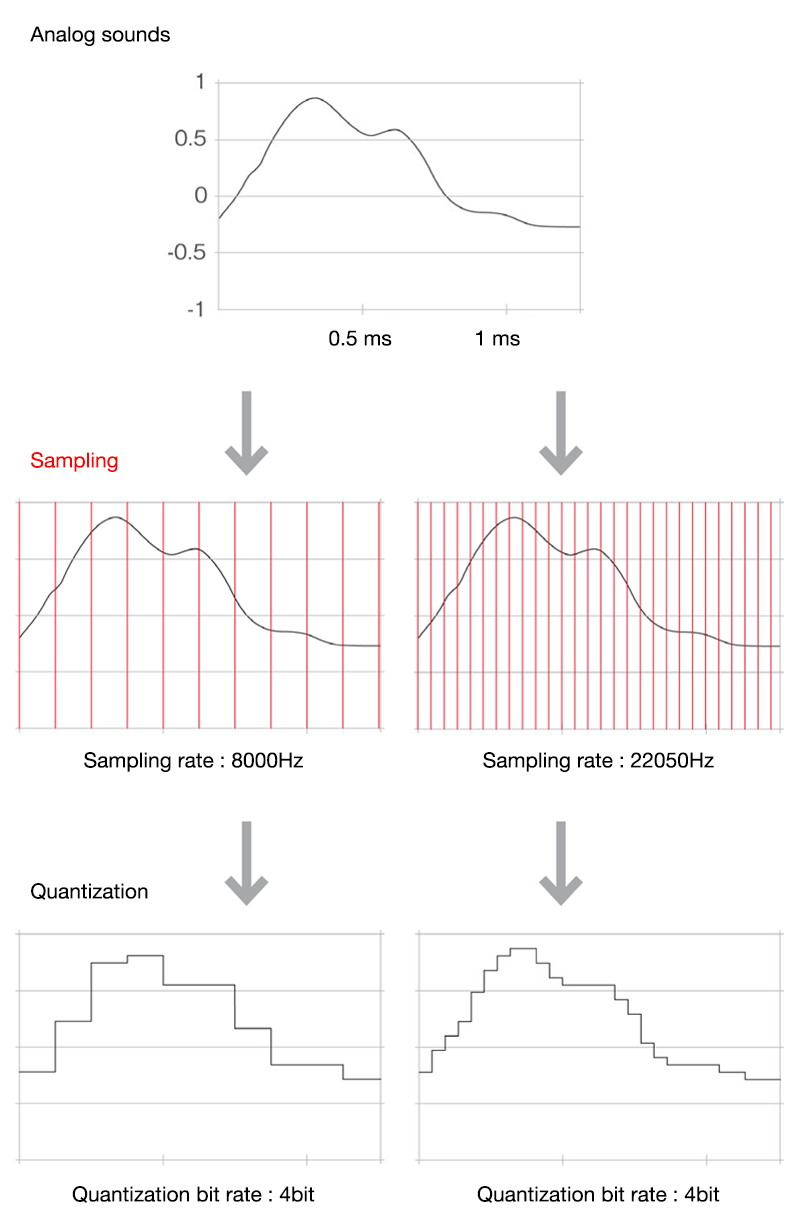# Sampling

Hyouhonka

## CATEGORIES

The process of converting analog data such as images and audio into digital data is called analog-digital (A-D) conversion. A-D conversion is performed via two processes: sampling and quantization. Sampling is the process of measuring continuously changing analog information at regular intervals.

With images, the colors of an analog image change continuously. To generate a bit map data (i.e. a digital image) out of this, one must first decide how finely the color values are to be measured. Sampling is thus the process of deciding this fineness and measuring the color values. Since a bit map data is a collection of pixels, when sampling, the analog image is divided into a grid pattern and a decision is made on the scope of a single pixel. The smaller the grids, the more faithfully the analog image is duplicated. (The data size increases in proportion.) When digitizing an image, sampling accuracy is determined by the input resolution. An input resolution of 300dpi or 350dpi is used for ordinary printing. This means that each inch of the analog image was divided up into 300 or 350 grids, with each grid constituting a pixel.

With sounds, analog sounds are waves propagating through a medium. To make digital data out of continuously changing sound waves, one must decide on the frequency for measuring those waves. Sampling is thus a process of deciding on that frequency (number of measurements per second) and actually measuring the sound wave values. The more frequently the sound is measured in the space of one second, the more faithfully the analog waves are reproduced. (The data size increases in proportion.) When digitizing sound, sampling accuracy is determined by the sampling rate. For example, music CDs use a sampling rate of 44,100Hz. This means that the analog sound is measured 44,100 times per second.

In this way, it is best to visualize analog images and sounds being converted into digital data via the same processes.

• Concepts of image sampling and quantization• Concepts of audio sampling and quantization